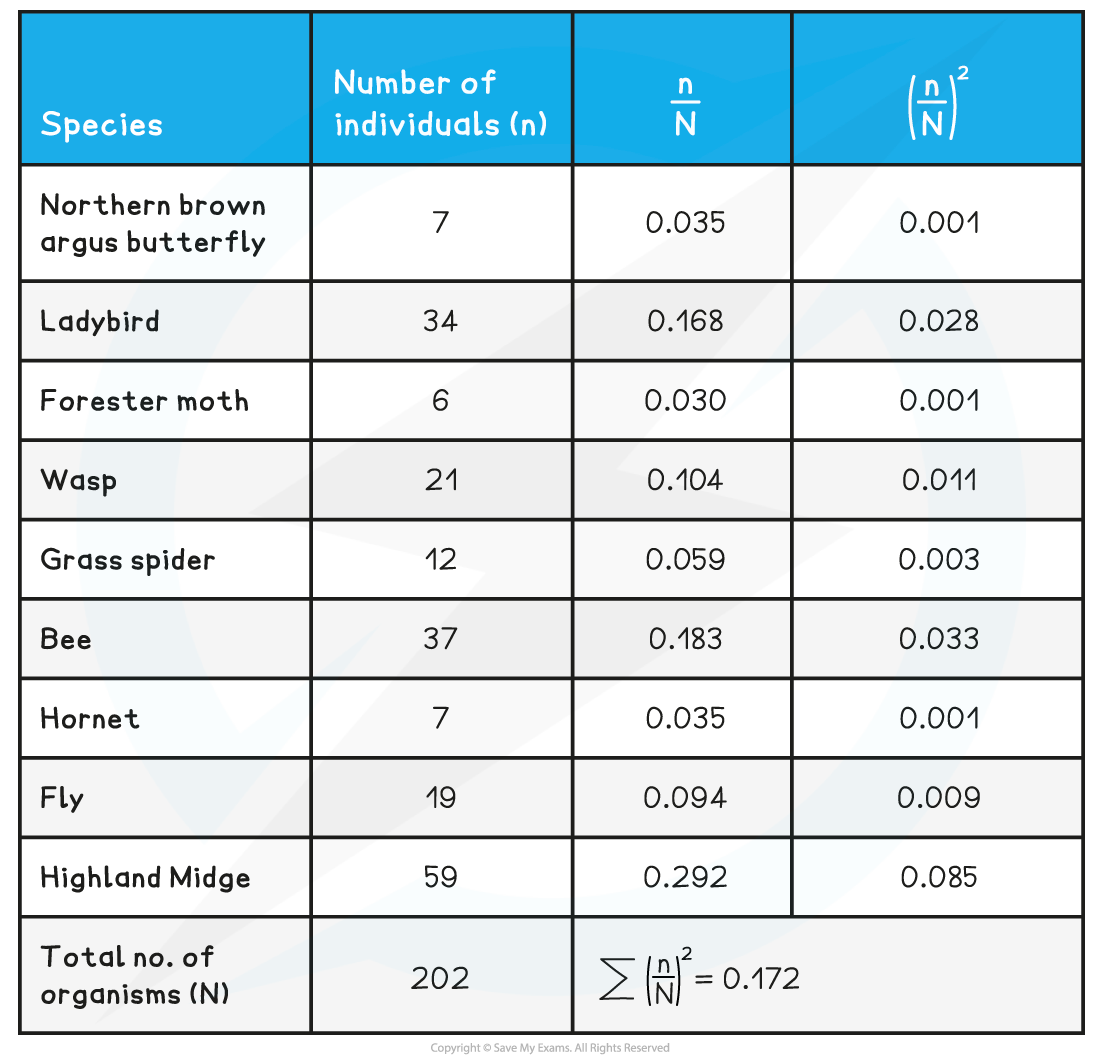# CIE A Level Biology复习笔记18.2.7 Simpson's Index

### Simpson's Index

• Once the abundance of different species in an area has been recorded the results can be used to calculate the species diversity or biodiversity for that area
• Species diversity looks at the number of different species in an area but also the evenness of abundance across the different species
• Simpson’s index of diversity (D) can be used to quantify the biodiversity of an area

#### Simpson's index

• The formula is:•  Where:
• n = total no. of organisms for a single species
• N = total no. of organisms for all species
• To calculate Simpson’s Index:
• Step 1: First step is to calculate n / N for each species
• Step 2: Square each of these values
• Step 3: Add them together and subtract the total from 1
• The possible values of D are significant:
• The value of D can fall between 0 and 1
• Values near 1 indicate high levels of biodiversity
• Values near 0 indicate low levels of biodiversity

#### Worked Example

Samples of different insect species in a back garden were collected using sweep nets and identification keys. Use the data to calculate Simpson’s Index.

The results and working out are seen in the table below. The figures have been rounded to three decimal places for columns 3 and 4D = 1 - 0.172 = 0.828

As the value of D is much closer to 1 than 0, it can be said that this is a relatively high value for biodiversity.

#### Exam Tip

Remember, you will be provided with the formula for Simpson’s Index in the exam. You need to be able to carry out the calculation to test for correlation, as you could be asked to do this in the exam. This also means you should understand when it is appropriate to use the different statistical tests, and the conditions in which each is valid.